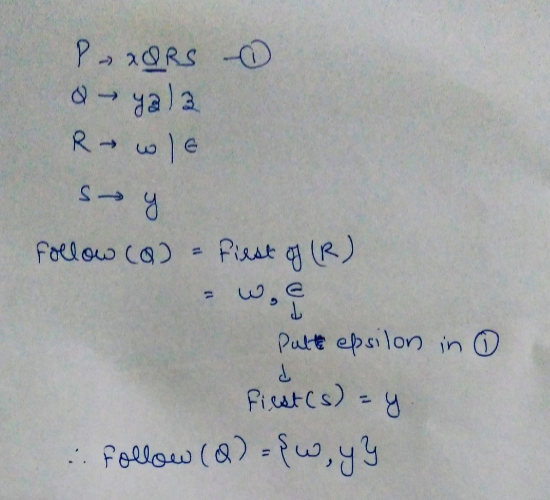Related Articles
GATE | GATE-CS-2017 (Set 1) | Question 23
• Last Updated : 20 Feb, 2017

Consider the following grammar

```p --> xQRS
Q --> yz|z
R --> w|∈
S -> y
```

Which is FOLLOW(Q)?

(A) {R}
(B) {w}
(C) {w, y}
(D) {w, ∉}

Answer: (C)

Explanation:Alternate Solution

To compute FOLLOW(A) for all non terminals A, apply the following rules until nothing can be added to any FOLLOW set:

• Place \$ in FOLLOW(S), where S is the start symbol and \$ is the input right end marker.
• If there is a production A → αBβ, then everything in FIRST(β), except for ∈, is placed in FOLLOW(B)
• If there is a production A → αB, or a production A → αBβ where FIRST(β) contains ∈ (i.e., b → ∈), then everything in FOLLOW(A) is in FOLLOW(B).

Therefore, follow(Q) = First(RS) = ( w ) ∪ First(S) = { w } ∪ { y } = { w,y }.

Note that here, first(S) is not ∈.

This explanation has been contributed by Mithlesh Upadhyay.

Quiz of this QuestionMy Personal Notes arrow_drop_up